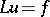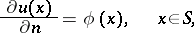# Neumann boundary conditions

(diff) ← Older revision | Latest revision (diff) | Newer revision → (diff)

Neumann conditions, Neumann data, boundary conditions of the second kind

Consider a second-order partial differential equationon a domaininwith boundary(cf. also Differential equation, partial, of the second order). Boundary conditions of the typewhereis the outward pointing normal at, are called Neumann boundary conditions.

A boundary value problem with Neumann conditions is also called a boundary value problem of the second kind (see Second boundary value problem).

How to Cite This Entry:
Neumann boundary conditions. Encyclopedia of Mathematics. URL: http://encyclopediaofmath.org/index.php?title=Neumann_boundary_conditions&oldid=18697
This article was adapted from an original article by M. Hazewinkel (originator), which appeared in Encyclopedia of Mathematics - ISBN 1402006098. See original article